Question

# If three lightbulbs with the negligible resistances are in series, would the first bulb in the...

If three lightbulbs with the negligible resistances are in series, would the first bulb in the circuit be the brightest? Why?

When three light bulbs are in series connection, then all light bulbs will shine with the same brightness because the amount of current is same through any component in a series connection.

==========

#### Earn Coins

Coins can be redeemed for fabulous gifts.

Similar Homework Help Questions
• ### Three 50-Ω lightbulbs and three 60-Ω lightbulbs are connected in series. What is the total resistance...

Three 50-Ω lightbulbs and three 60-Ω lightbulbs are connected in series. What is the total resistance of the circuit? What is the total resistance if all six are wired in parallel?

• ### Three 30-12 lightbulbs and three 75-82 lightbulbs are connected in series. What is the total resistance...Three 30-12 lightbulbs and three 75-82 lightbulbs are connected in series. What is the total resistance of the circuit? Express your answer to two significant figures and include the appropriate units. HA ? Rtotal Value Omega Submit Request Answer Part B What is the total resistance if all six are wired in parallel? Express your answer to two significant figures and include the appropriate units. HA ? Rtotal = Value Omega

• ### Question 4 1.5 pts Three 38-2 lightbulbs and three 71-2 lightbulbs are connected in series. What...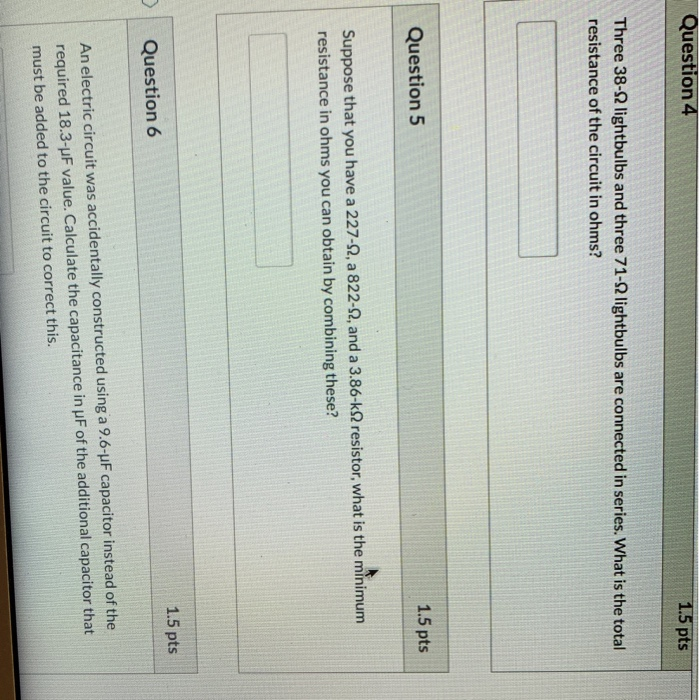Question 4 1.5 pts Three 38-2 lightbulbs and three 71-2 lightbulbs are connected in series. What is the total resistance of the circuit in ohms? Question 5 1.5 pts Suppose that you have a 227-2, a 822-12, and a 3.86-k2 resistor, what is the minimum resistance in ohms you can obtain by combining these? 1.5 pts Question 6 An electric circuit was accidentally constructed using a 9.6-4F capacitor instead of the required 18.3-uF value. Calculate the capacitance in uF of...

• ### Constar Three 40-N2 lightbulbs and three 60-22 lightbulbs are connected in series. Part A What is...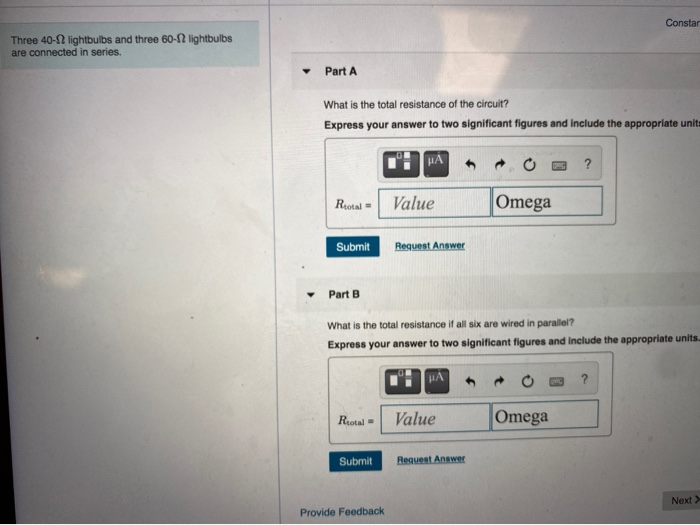Constar Three 40-N2 lightbulbs and three 60-22 lightbulbs are connected in series. Part A What is the total resistance of the circuit? Express your answer to two significant figures and include the appropriate unit MA ? Rotal Value Omega Submit Request Answer Part B What is the total resistance if all six are wired in parallel? Express your answer to two significant figures and include the appropriate units. HA Riotal Value Omega Submit Request Answer Next > Provide Feedback

• ### If two light bulbs are set up in parallel and one in series as shown in...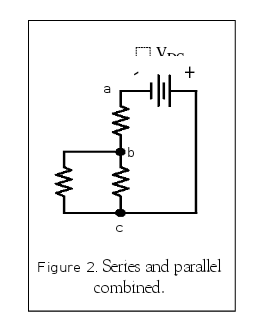If two light bulbs are set up in parallel and one in series as shown in the circuit above which bulb would be the brightest and why? Figure 2 Series and parallel combined

• ### Identical batteries with negligible internal resistance are connected in different arrangements to the same light bulb,...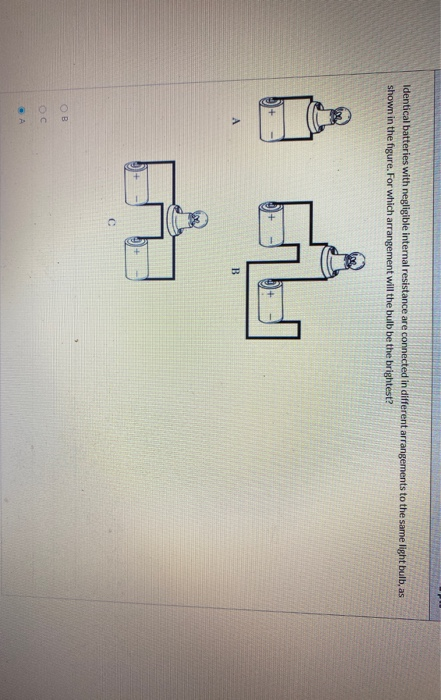Identical batteries with negligible internal resistance are connected in different arrangements to the same light bulb, as shown in the figure. For which arrangement will the bulb be the brightest? On A B C OB

• ### 5. Rank the order for how brightly the bulb would shine in the following circuits, with...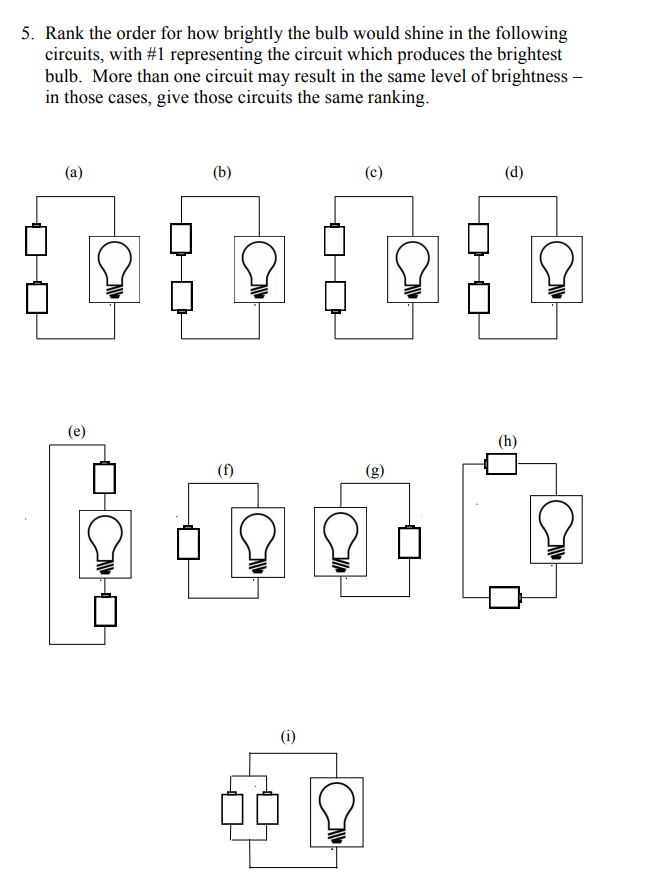5. Rank the order for how brightly the bulb would shine in the following circuits, with #1 representing the circuit which produces the brightest bulb. More than one circuit may result in the same level of brightness- in those cases, give those circuits the same ranking.

• ### 18 1 point The circuit below consists of three identical lightbulbs, a battery, and a switch....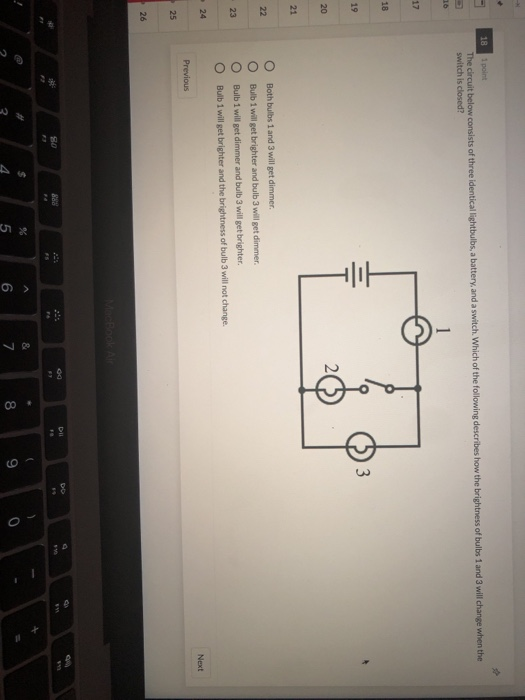18 1 point The circuit below consists of three identical lightbulbs, a battery, and a switch. Which of the following describes how the brightness of bulbs 1 and 3 will change when the switch is closed? 18 19 O O O O Both bulbs 1 and 3 will get dimmer. Bulb 1 will get brighter and bulb 3 will get dimmer. Bulb 1 will get dimmer and bulb 3 will get brighter. Bulb 1 will get brighter and the brightness...

• ### Two 75.0 W (120 V) lightbulbs are wired in series, then the combination is connected to...

Two 75.0 W (120 V) lightbulbs are wired in series, then the combination is connected to a 230 V supply. How much power is dissipated by each bulb? Answer in W.

• ### PSS 26.1 Resistors in Series and Parallel Learning Goal: To practice Problem-Solving Strategy 26.1 Resistors in...PSS 26.1 Resistors in Series and Parallel Learning Goal: To practice Problem-Solving Strategy 26.1 Resistors in Series and Parallel. Part A Two bulbs are connected in parallel across a source of emf = 12.0 V with a negligible internal resistance. One bulb has a resistance of 3.0 2, and the other is 3.5 . A resistor R is connected in Which of the following circuit diagrams represents the circuit described in this problem? A the circuit in series with the...